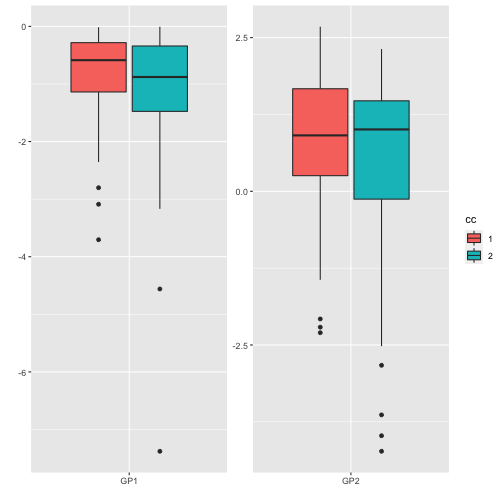# Plotting glycans with glycanr package

First we need to load the package.

``````# load glycanr package
library(glycanr)
``````
``````## From version 0.3 functions tanorm and glyco.outliers expect data frames
## in long format.
``````

Lets create a data.frame to simulate our glycan data.

``````set.seed(123)
n <- 200
X <- data.frame(ID=1:n, GP1=runif(n), GP2=rexp(n, 0.3),
GP3=rgamma(n, 2), cc=factor(sample(1:2, n, replace=TRUE)))
``````

Now we have data.frame X where GP represents glycans, ID represents e.g. sample IDs and cc represents Case/Control status.

``````head(X)
``````
``````##   ID       GP1       GP2       GP3 cc
## 1  1 0.2875775 6.0104311 2.4551743  1
## 2  2 0.7883051 0.1001982 0.2778425  1
## 3  3 0.4089769 4.3448018 1.1637760  2
## 4  4 0.8830174 0.6659741 1.7217277  2
## 5  5 0.9404673 5.8381875 1.5926956  1
## 6  6 0.0455565 5.8788944 2.0702267  1
``````

This data can now be plotted with glyco.plot function.

Basic usage is given as follows.

``````glyco.plot(X)
``````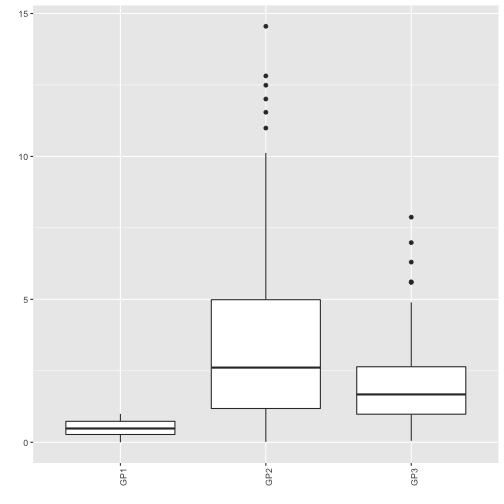It plots boxplots for every column whose name starts with 'GP'. To change boxplots with violin plots (represents the density of a data) option violin should be used.

``````glyco.plot(X, violin=TRUE)
``````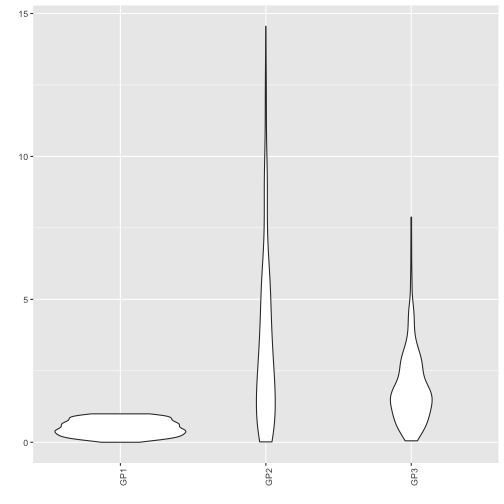To separate boxplots (or violin plots) into different layers option collapse should be used.

``````glyco.plot(X, collapse=FALSE)
``````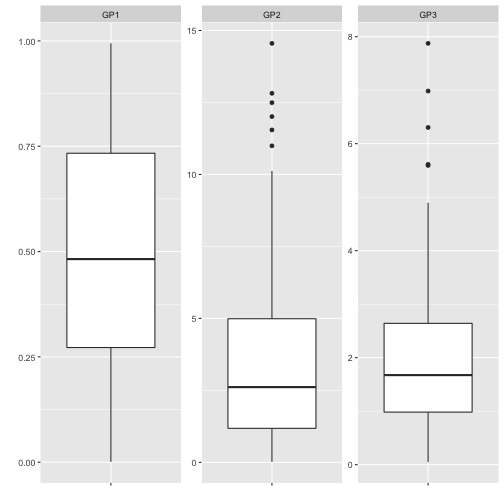To plot log transformed data option log.transform should be used

``````glyco.plot(X, collapse=FALSE, log.transform=TRUE)
``````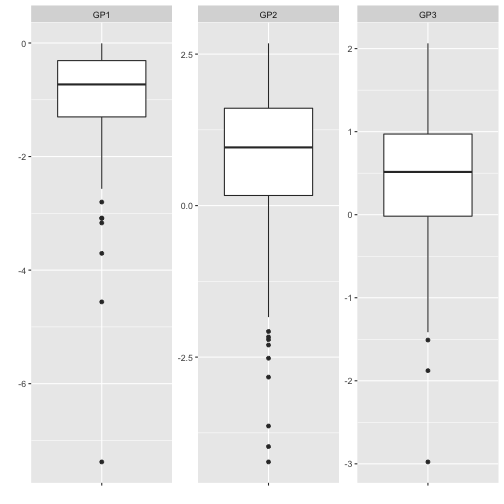### Grouping

If you want to see the difference between groups with boxplots (or violin plots) option group should be used. It takes a character string representing the name of the column on grouping should be done.

Grouping by cc variable can be done like this.

``````glyco.plot(X, collapse=FALSE, log.transform=TRUE, group="cc")
``````
``````## \$p.val.unadj
##        GP1        GP2        GP3
## 0.07474019 0.20680798 0.95699301
##
## \$p.val
##       GP1       GP2       GP3
## 0.2242206 0.4136160 0.9569930
##
## \$plot
``````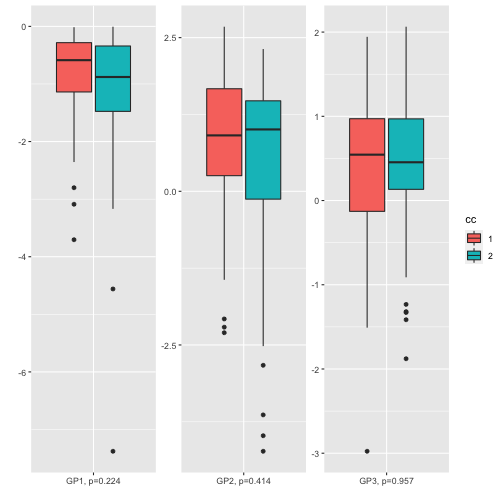As it can be seen the default option is to also conduct a test in difference between groups (Mann-Whitney-Wilcoxon for 2 groups, Kruskal-Wallis for more groups) and print the obtained p-values. The printed values are corrected for multiple testing before printing. As the output you get original p-values and adjusted p-values together with the plot.

``````glyco.plot(X, collapse=FALSE, log.transform=TRUE, group="cc", p.adjust.method="fdr")
``````
``````## \$p.val.unadj
##        GP1        GP2        GP3
## 0.07474019 0.20680798 0.95699301
##
## \$p.val
##       GP1       GP2       GP3
## 0.2242206 0.3102120 0.9569930
##
## \$plot
``````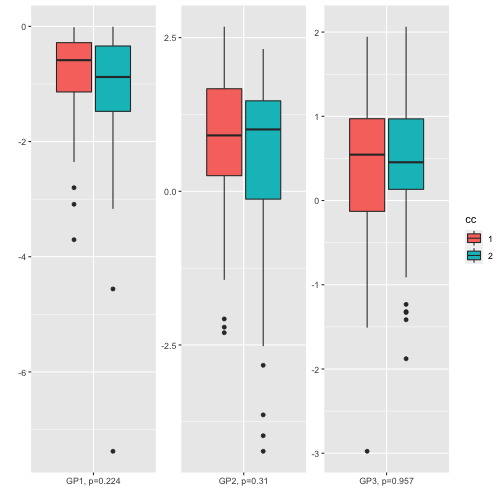Printing p-values in plots can be omitted with print.p.values parameter.

``````glyco.plot(X, collapse=FALSE, log.transform=TRUE, group="cc", p.adjust.method="fdr",
print.p.values=FALSE)
``````
``````## \$p.val.unadj
##        GP1        GP2        GP3
## 0.07474019 0.20680798 0.95699301
##
## \$p.val
##       GP1       GP2       GP3
## 0.2242206 0.3102120 0.9569930
##
## \$plot
``````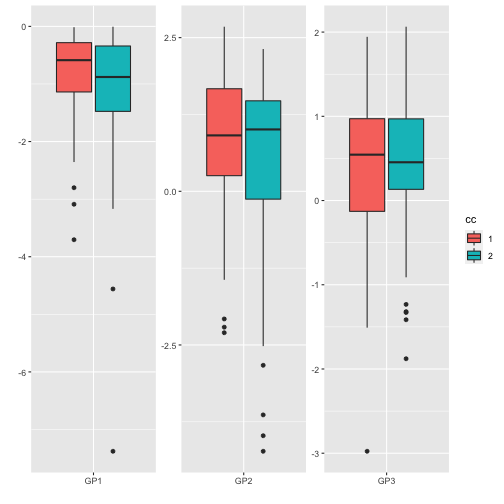When grouping, by default, all glycans are plotted. To plot only those that differ statistically significant parameter all should be used.

## Choosing other columns

Function glyco.plot plots all columns whose name starts with GP. Since these plotting techniques can be used on other data as well there is the parameter glyco.names to choose which columns you want to use.

``````glyco.plot(X, collapse=FALSE, log.transform=TRUE, group="cc", p.adjust.method="fdr",
print.p.values=FALSE, glyco.names=c("GP1", "GP2"))
``````
``````## \$p.val.unadj
##        GP1        GP2
## 0.07474019 0.20680798
##
## \$p.val
##       GP1       GP2
## 0.1494804 0.2068080
##
## \$plot
``````